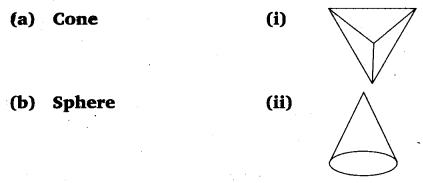# Class 6 Maths NCERT Solutions for Chapter 5 Understanding Elementary Shapes Ex – 5.9

## Understanding Elementary Shapes

Question 1.
Match the following:Give two new examples of each shape.

Solution:
Matching is as under:
(a) → (ii)
(b) → (iv)
(c) → (v)
(d) → (iii)
(e) → (i)

Two new examples of

(a) Cone: Conical tent.
(b) Sphere: Tennis ball, a ball of wool.
(c) Cylinder: Measuring jars, gas cylinder.
(d) Cuboid: Brick, Match box.
(e) Pyramid: Pyramids of Egypt.

Question 2.
What shape is

(b) A brick?
(c) A match box?

Solution:
(a) Cuboid
(b) Cuboid
(c) Cuboid
(d) Cylinder
(e) Sphere